# Test: Laplace Transform- 3

## 10 Questions MCQ Test GATE ECE (Electronics) 2023 Mock Test Series | Test: Laplace Transform- 3

Description
Attempt Test: Laplace Transform- 3 | 10 questions in 30 minutes | Mock test for Electronics and Communication Engineering (ECE) preparation | Free important questions MCQ to study GATE ECE (Electronics) 2023 Mock Test Series for Electronics and Communication Engineering (ECE) Exam | Download free PDF with solutions
QUESTION: 1

### Determine the time signal x(t) corresponding to given X (s) and choose correct option. Q.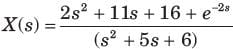Solution: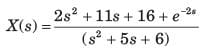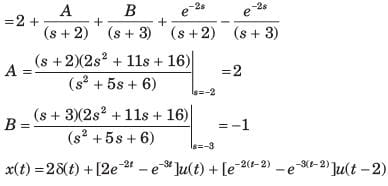QUESTION: 2

### Determine the time signal x(t) corresponding to given X (s) and choose correct option. Q.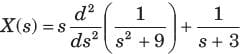Solution: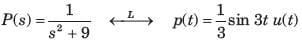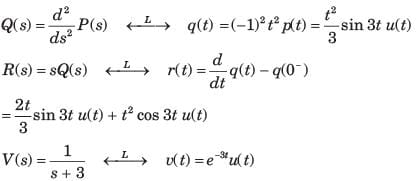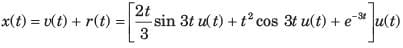QUESTION: 3

### Determine the time signal x(t) corresponding to given X (s) and choose correct option. Q.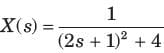Solution: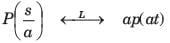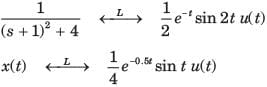QUESTION: 4

Determine the time signal x(t) corresponding to given X (s) and choose correct option.
Q.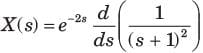Solution: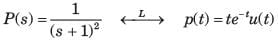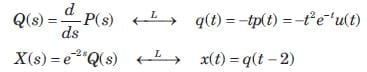x(t) =  -( t - 2) 2e-(t-2) u( t - 2)

QUESTION: 5

Given the transform pair below. Determine the time signal y(t) and choose correct option.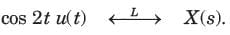Q. Y (s) = ( s + 1) X ( s)

Solution: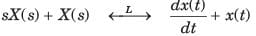⇒ y(t) = ( -2 sin2 t + cos 2 t ) u( t)

QUESTION: 6

Given the transform pair below. Determine the time signal y(t) and choose correct option.Q. Y (s) = X ( 3s)

Solution: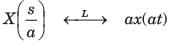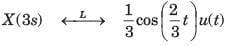QUESTION: 7

Given the transform pair below. Determine the time signal y(t) and choose correct option.Q. Y (s) = X ( s + 2)

Solution: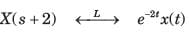QUESTION: 8

Given the transform pair below. Determine the time signal y(t) and choose correct option.Q.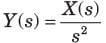Solution: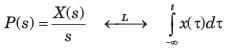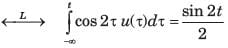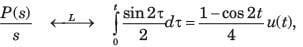QUESTION: 9

Given the transform pair below. Determine the time signal y(t) and choose correct option.Q.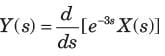Solution: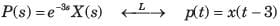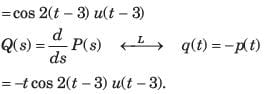QUESTION: 10

Given the transform pair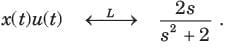Determine the Laplace transform Y (s) of the given time signal in question and choose correct option.
Q. y(t) = x(t - 2)

Solution: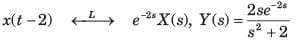Use Code STAYHOME200 and get INR 200 additional OFF Use Coupon Code